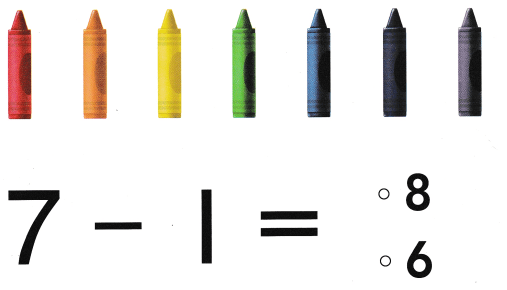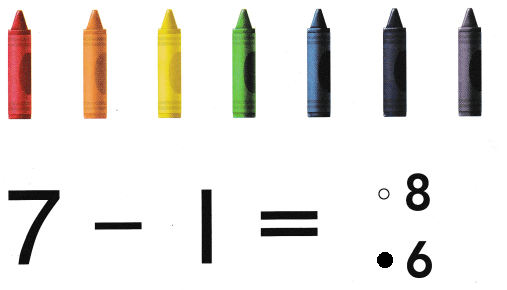# Texas Go Math Kindergarten Lesson 13.1 Answer Key One More and One Less

Refer to our Texas Go Math Kindergarten Answer Key Pdf to score good marks in the exams. Test yourself by practicing the problems from Texas Go Math Kindergarten Lesson 13.1 Answer Key One More and One Less.

## Texas Go Math Kindergarten Lesson 13.1 Answer Key One More and One Less

Essential Question
How do you show one more and one less?

Explore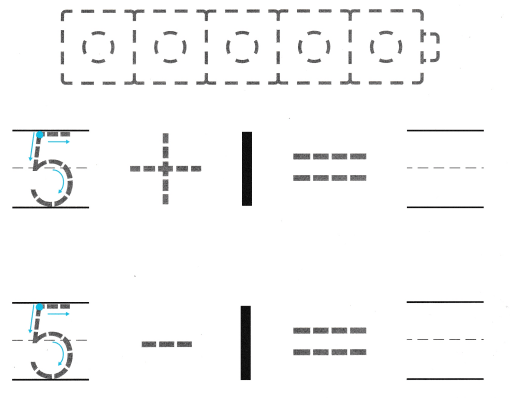Explanation:
5 blue cubes
and one red cube
5  + 1 = 6
5 – 1 = 4
so, 4 blue cubes

Directions
Place 5 blue cubes on the counter outline. Trace the number. Add 1 red counter. Write the number to show the sum. Place 5 blue cubes on the counter outline. Write the number. Take one counter away. Write the number to show the difference.

Share and Show

Question 1.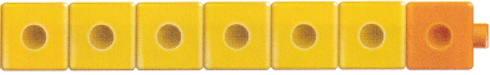Explanation:
Number of yellow counters 6
number of orange counters 1
Total number of counters 7
6 + 1 = 7

Question 2.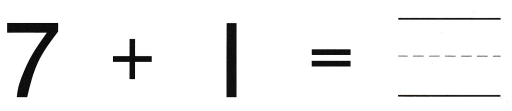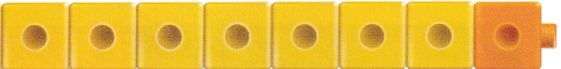Explanation:
Number of yellow counters 7
number of orange counters 1
Total number of counters 8

Question 3.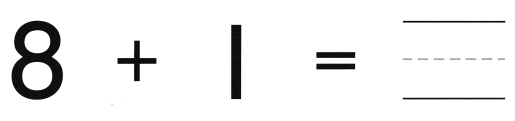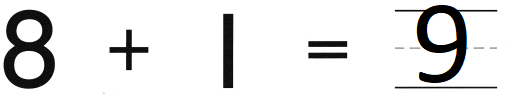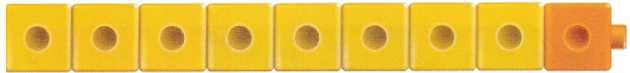Explanation:
Number of yellow counters 8
number of orange counters 1
Total number of counters 9

Question 4.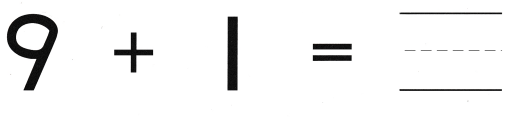Explanation:
Number of yellow counters 9
number of orange counters 1
Total number of counters 10

Directions
1-4. Use two colors of cubes to show the sum. Write the number.

Question 5.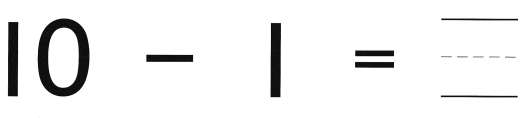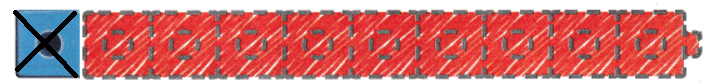Explanation:
There are 10 cubes 9 cubes are red and 1 cube of blue
so, removed blue cube
10 – 1 = 9

Question 6.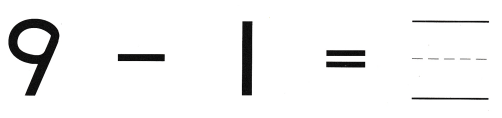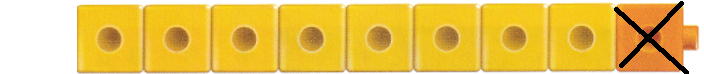Explanation:
The difference of 9 and 1 is 8

Question 7.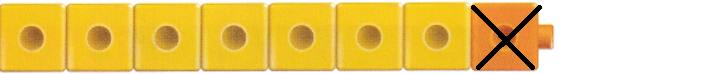Explanation:
The difference of 8 and 1 is 7
8 – 1 = 7

Question 8.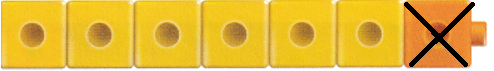Explanation:
The difference of 7 and 1 is 6
7 – 1 = 6

Directions
5-8. Use two colors of cubes to show the difference. Write the number.

Home Activity

• Show your child a set of 5 to 10 toys. Have your child create a set of objects that shows one more and one less.
Explanation: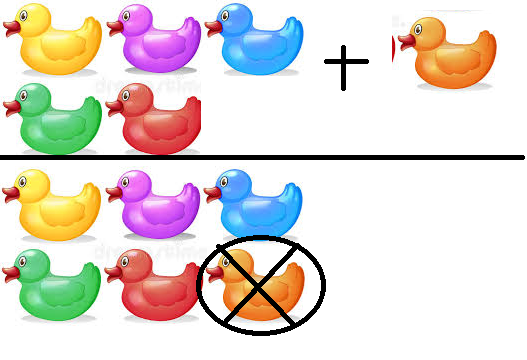5 + 1 = 6
a group of 5 ducks 1 more is added to it
6 – 1 = 4
a group of 6 ducks 1 is removed from it

Problem Solving

Question 9.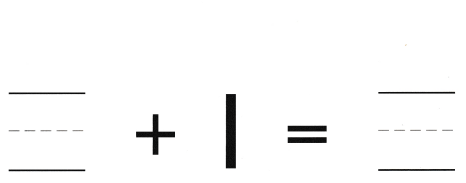Explanation:
The sum of 5 and 1 is 6
5 + 1 = 6

Question 10.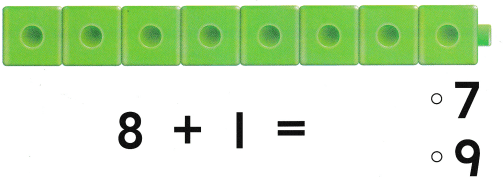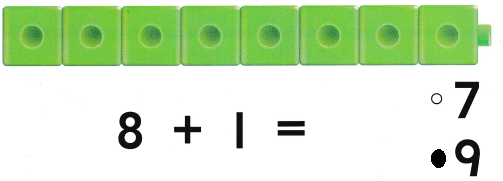Explanation:
The sum of 8 and 1 is 9
8 + 1 = 9
Lanie has 9 cubes now

Directions
9. Wesley has 5 marbles. Draw to show how many marbles Wesley has. Write the number. Annie has 1 more marble than Wesley. How many marbles does Annie have? Write the number. 10. Choose the correct answer. Lanie has 8 cubes. What number is one more?

### Texas Go Math Kindergarten Lesson 13.1 Homework and Practice

Question 1.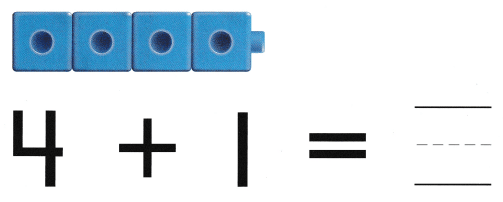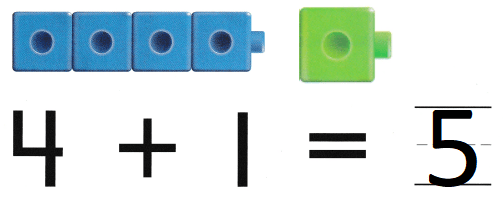Explanation:
the sum of 4 and 1 is 5
4 + 1 = 5

Question 2.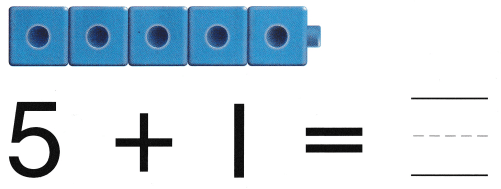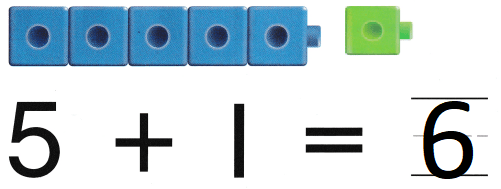Explanation:
The sum of 1 and 5 is 6
5 + 1 = 6

Directions
1-2. Draw one more cube to show the sum. Use a different color. Write the sum.

Texas Test Prep

Lesson Check

Question 3.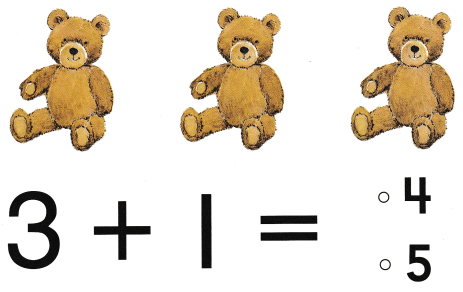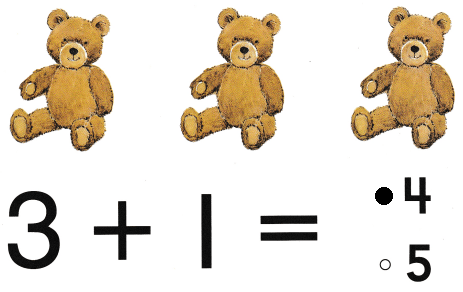Explanation:
Selena has 3 brown toy bears
1 more bear is 4
3 + 1 = 4

Question 4.